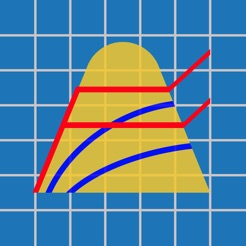•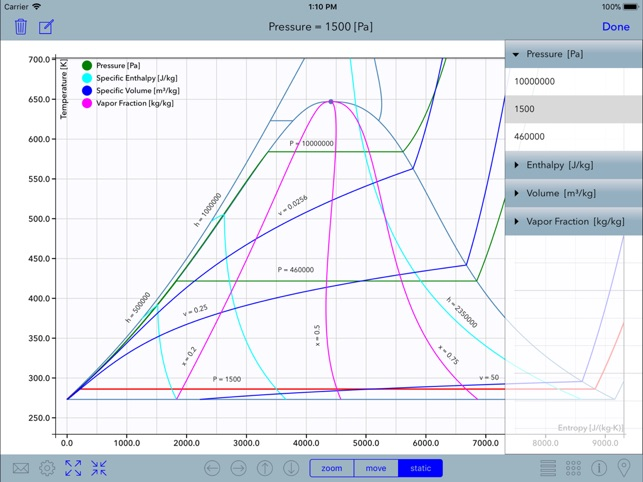••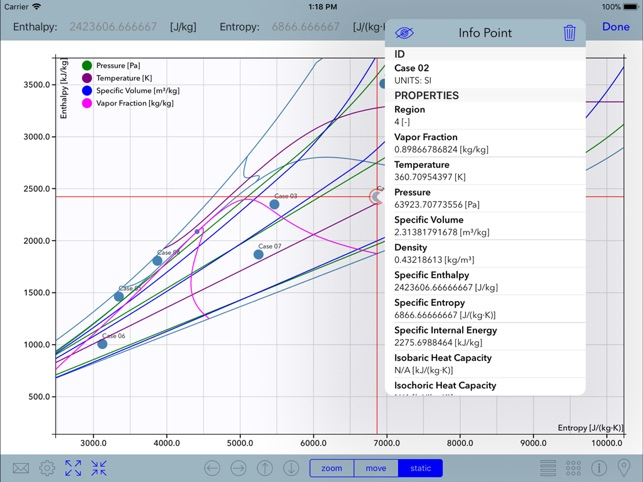•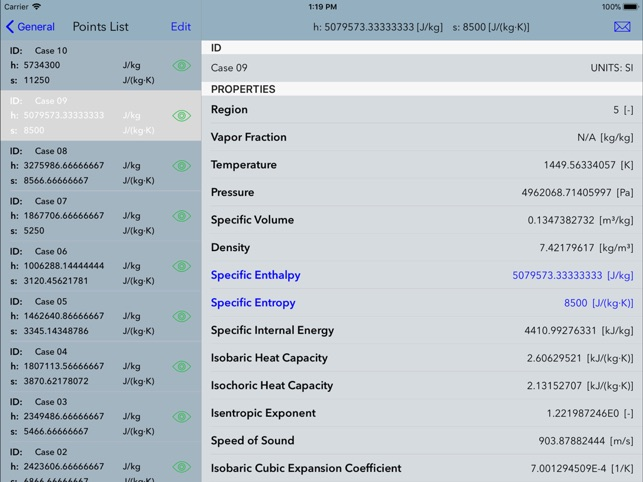•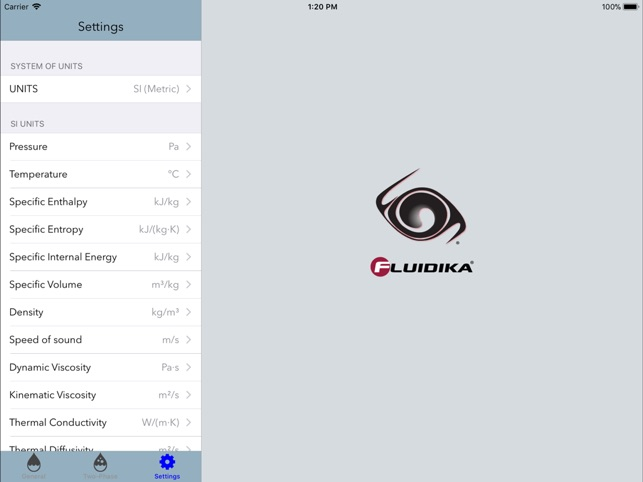Description

Steam Plot performs calculations and generates diagram plots of thermodynamic and transport properties of steam based on the latest IAPWS-IF97 Formulation and IAPWS releases.
Given a combination of two thermodynamic properties out of 14 different possible combinations, it calculates 20 thermodynamic and transport properties of steam and 10 partial derivatives.
Calculates and plots isolines of pressure, temperature, specific enthalpy, specific entropy, specific volume and vapor fraction for 4 different diagram plots.
The numerical results and diagram plots obtained are suitable for engineering, scientific, industrial or academic use.

-Performs all the calculations implementing the latest mathematical formulations from the IAPWS (International Association for the Properties of Water and Steam), IAPWS-IF97 Industrial formulation (Revision 2007).

The following applies when the application has enabled the Full Range of Variables:

-Calculates 20 Thermodynamic and Transport properties of steam using the above formulation:
Pressure
Temperature
Specific Enthalpy
Specific Entropy
Specific Internal Energy
Specific Volume
Density
Speed of Sound
Dynamic Viscosity
Kinematic Viscosity
Thermal Conductivity
Thermal Diffusivity
Isobaric Heat Capacity
Isochoric Heat Capacity
Isentropic Exponent
Isobaric Cubic Expansion Coefficient
Isothermal Compressibility
Prandtl Number
Vapor Fraction
IAPWS-IF97 Region

-Calculates 10 partial derivatives:
Derivate of specific volume on pressure with constant temperature.
Derivate of specific internal energy on pressure with constant temperature.
Derivate of specific entropy on pressure with constant temperature.
Derivate of specific enthalpy on pressure with constant temperature.
Derivate of specific volume on temperature with constant pressure.
Derivate of specific internal energy on temperature with constant pressure.
Derivate of specific entropy on temperature with constant pressure.
Derivate of specific enthalpy on temperature with constant pressure.
Derivate of pressure on temperature with constant specific volume.
Derivate of pressure on specific volume with constant temperature.

-Allows for 14 different combinations of thermodynamic properties to be entered as input parameters:
Temperature / Pressure
Temperature / Enthalpy ... etc

-The calculations operate in two modes denoted as General Properies and Two-Phase Properties:
General Properties: Input and Output values are all over the range of the IAPWS-IF97 Formulation.
Two-Phase Properties: Input and output thermodynamics property values are on the range of the two-phase region (region 4) of the IAPWS-IF97 Formulation, including saturation values of liquid and vapor.

-Supports input parameters and calculation results in both the SI (metric) and the I-P (English) system of units.

-For each combination of input thermodynamic properties, it calculates and provides the user with information about the appropriate input values in the valid range of calculations.

-Results can be sent by email in an HTML file along with a comma-separated value (CSV) file.

-Generates 4 Diagram Plot types with isolines calculated all over the IAPWS-IF97 range:
Temperature - Entropy :
Pressure
Specific enthalpy
Specific volume
Vapor Fraction

Enthalpy - Entropy :
Pressure
Temperature
Specific volume
Vapor Fraction

log Pressure - Enthalpy :
Temperature
Specific entropy
Specific volume
Vapor fraction

Pressure - Temperature :
Specific enthalpy
Specific entropy
Specifc volume

- Steam points calculated from General or Two-Phase properties can be stored in a database for later retrieval.

- Plot diagrams projects allow to add and edit isolines and steam calculation points graphically or numerically directly to plot diagrams that can be saved into a database.

-Plot diagrams can be sent by email as high-resolution PDF fles.

What’s New

Version 2.1

-Update to iOS 12.1.

Information

Seller
Size
28.8 MB
Category
Productivity
Compatibility

Requires iOS 12.1 or later. Compatible with iPad.

Languages

English

Age Rating
Rated 4+
© 2019 Fluidika Techlabs, S de RL de CV
Price
Free
In-App Purchases
1. Full Range of Variables \$49.99

Supports

•